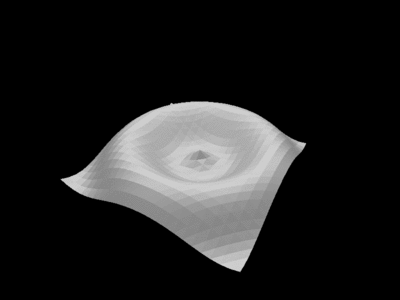One of the concepts behind Linux (or Unix) design is to execute small programs which process each others outputs to deliver final result. In this article I will present how to create a small animation which uses several Linux utilities.## Wave

Animation uses well know POV-Ray raytracing tool to generate 3D `z = sin(x, y)` function. First we need to generate a frame of the animation sequence.

``````record Point
{
x : float;
y : float;
z : float;
}

func print_point(p : Point) -> int
{
prints("<" + p.x + ", " + p.y + ", " + p.z + ">");
0
}

func print_rect(data[D1, D2] : Point, x : int, y : int) -> int
{
prints("triangle { ");
print_point(data[x, y]);
prints(", ");
print_point(data[x + 1, y]);
prints(", ");
print_point(data[x + 1, y + 1]);
prints(" }\n");

prints("triangle { ");
print_point(data[x, y]);
prints(", ");
print_point(data[x + 1, y + 1]);
prints(", ");
print_point(data[x, y + 1]);
prints(" }\n");

0
}

func print_wave(data[D1, D2] : Point) -> int
{
var x = 0;
var y = 0;

for (x = 0; x < D1 - 1; x = x + 1)
{
for (y = 0; y < D2 - 1; y = y + 1)
{
print_rect(data, x, y)
}
};

0
}

{
prints("\
camera { \
location <0, -16, -12> \
look_at <0, 0, 0> \
rotate <0, 0, 30> \
} \
light_source { <0, 0, -32> color rgb<1, 1, 1> } \
mesh { \n");

0
}

func tail() -> int
{
prints("\
texture { \
pigment { color rgb<0.9, 0.9, 0.9> } \
finish { ambient 0.2 diffuse 0.7 } \
} \
}\n");

0
}

func get_wave(phi : float, size : int) -> [_, _] : Point
{
var x = 0;
var y = 0;
var x_v = 0.0;
var y_v = 0.0;
var z_v = 0.0;
var tab = {[ size, size ]} : Point;

for (x = 0; x < size; x = x + 1)
{
for (y = 0; y < size; y = y + 1)
{
tab[x, y] = Point(0.0, 0.0, 0.0);

x_v = -2.0 * 3.141 + 4.0 * 3.141 / size * x;
y_v = -2.0 * 3.141 + 4.0 * 3.141 / size * y;
z_v = sin(phi + sqrt(x_v * x_v + y_v * y_v));

tab[x, y].x = x_v;
tab[x, y].y = y_v;
tab[x, y].z = z_v
}
};

tab
}

func generate(phi : float) -> int
{
var data = get_wave(phi, 24);

print_wave(data);
tail();

10
}

func main(phi : int) -> int
{
generate(2.0 * 3.141 / 32.0 * phi);
0
}

``````

The above listsing uses Never programming language to generate Povray scene description. 3D data is calculated in function `get_wave`. Sin function values range from -2pi to 2pi both for `x` and `y` arguments. Next the data is output as Povray mesh by functions `head`, `print_wave` and `tail`. Function `main` takes an argument which specifies frame number. Frame number is an animation offset used in `sin` function.

## Povray, bash and convert

To generate animation several frames need to be combined together.

``````for f in {0..63}
do
never -m 50000 -f wave.nev \$f > wave_\$f.pov
done
``````

First 64 frames are generated which pass offset from 0 to 4*pi which gives full animation cycle.

Next frames are processed with Povray to generate graphics.

``````for f in wave*.pov
do
povray \$f
done
``````

And last they are combined together with convert tool.

``````convert -delay 10 -loop 0 wave_{0..63}.png wave.gif
``````

I hope you liked this tutorial!